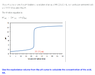# Calculating Concentration of Acid using a pH Titration Curve

• Chemistry
• Justinboln

#### Justinboln

Homework Statement
calculate the concentration of the acid, HA.
Relevant Equations
not too sure
Use the equivalence volume from the pH curve to calculate the concentration of the acid, HA.

I'm not sure which equation to use or how to approach this question (Attached).

Thank you!

#### Attachments

•Question.png
10.3 KB · Views: 166
Hint: this is a simple stoichiometry (combined with n=CV). Titration curve is just a way of finding out when the amount of added titrant was equivalent to the amount of the acid present.

•Justinboln